主頁 高登熱話 吃喝玩樂 科技消費 名人專訪 短片現有會員可[按此]登入。未成為會員可[按此]註冊。 [公司模式 - 關]  [懷舊模式 - 開]  [Youtube 預覽 - 關]  [大字型]  [小字型] 您現在聚腳在 學術台內。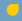高登公告 有關整合forum 1~15事宜 高登討論區定於下星期三（14/6）將forum1~15整合為http://forum.hkgolden.com，屆時有部分未獲授權的第三方app與瀏覽器plugin或會受到影響。不便之處，敬請原諒。精選文章DeerGamer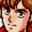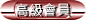If a,b are roots of quadratic equation Ax^2+Bx+C+D=0find a^4+b^4 if B=C+D.B^4-4B^3+2B^2 11/1/2020 18:13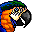If a,b are roots of quadratic equation Ax^2+Bx+C+D=0find a^4+b^4 if B=C+D.B^4-4B^3+2B^2step?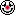11/1/2020 18:21雖然中學生都做到但係睇唔出邊到超簡單 11/1/2020 18:22If a,b are roots of quadratic equation Ax^2+Bx+C+D=0find a^4+b^4 if B=C+D.B^4-4B^3+2B^2step?岩唔岩先得架?11/1/2020 18:22If a,b are roots of quadratic equation Ax^2+Bx+C+D=0
find a^4+b^4 if B=C+D.

B^4-4B^3+2B^2

step?11/1/2020 18:23Since Ax^2+Bx+C+D=0 has real root 1ie A(1)^2+B(1)+C+D=0 (given)discriminant>=0B^2-4A(C+D)>=0---(1)Similarly, because (A+B)x^2+Cx+D has real roots 1C^2-4(A+B)D>=0---(2)(1)+(2): B^2+C^2-4(A(C+D)+(A+B)D)>=0B^2+C^2-4(AC+AD+AD+BD)>=0 11/1/2020 20:29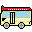For all x=0, put y=0We have f(x乘0）=f(x)f(0)f(0)=f(x)f(0)f(x)=1 11/1/2020 20:38For all x=0, put y=0
We have f(x乘0）=f(x)f(0)
f(0)=f(x)f(0)
f(x)=1

nice try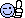11/1/2020 20:51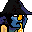Given that f(0) =/= 0, find all function(s) such that f(x)f(y)=f(xy) for any real numbers x,y.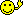f(x) = 1/xf(y) = 1/yf(xy) = 1/xy = f(x)f(y)搵到一個答案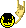11/1/2020 21:58Oh原來第一條有古惑f(x)=x^p for some real numbers pf(x)f(y)=(x^p)(y^p)=(xy)^p=f(xy) 11/1/2020 22:10f(x)=1係special case.

11/1/2020 22:11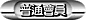Given that f(0) =/= 0, find all function(s) such that f(x)f(y)=f(xy) for any real numbers x,y.f(x) = 1/xf(y) = 1/yf(xy) = 1/xy = f(x)f(y)搵到一個答案應該唔得，因為0嘅時候係undefined不過如果幫0加exception嘅話，咁就無問題 11/1/2020 22:12最衰我無寫f(x) is defined for all real numbers x 11/1/2020 22:12Given that f(0) =/= 0, find all function(s) such that f(x)f(y)=f(xy) for any real numbers x,y.f(x) = 1/x
f(y) = 1/y
f(xy) = 1/xy = f(x)f(y)

11/1/2020 22:13最衰我無寫f(x) is defined for all real numbers x你題目寫for any real number x, y 其實implies咗f(x)一定要well-defined for all real number x 12/1/2020 00:29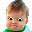If a,b are roots of quadratic equation Ax^2+Bx+C+D=0find a^4+b^4 if B=C+D.My instinct is to find an expression for a^4+b^4 in terms of ab and a+ba^4+b^4=(a+b)^4 - (4a^3)(b) - (6a^2)(b^2) - (4a)(b^3)=(a+b)^4 - 6(ab)^2 - 4(ab)(a^2+b^2)=(a+b)^4 - 6(ab)^2 - 4(ab)((a+b)^2-2ab))and substitute the corresponding coefficients Although there could be a quicker way. 12/1/2020 01:38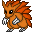function 有得find all 架咩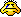12/1/2020 01:41Oh原來第一條有古惑
f(x)=x^p for some real numbers p
f(x)f(y)=(x^p)(y^p)=(xy)^p=f(xy)

but the question says f(0) is not 0

12/1/2020 01:42Given that f(0) =/= 0, find all function(s) such that f(x)f(y)=f(xy) for any real numbers x,y.f(x) = 1/xf(y) = 1/yf(xy) = 1/xy = f(x)f(y)搵到一個答案錯 12/1/2020 08:37If a,b are roots of quadratic equation Ax^2+Bx+C+D=0
find a^4+b^4 if B=C+D.

My instinct is to find an expression for a^4+b^4 in terms of ab and a+b

a^4+b^4
=(a+b)^4 - (4a^3)(b) - (6a^2)(b^2) - (4a)(b^3)
=(a+b)^4 - 6(ab)^2 - 4(ab)(a^2+b^2)
=(a+b)^4 - 6(ab)^2 - 4(ab)((a+b)^2-2ab))

and substitute the corresponding coefficients
Although there could be a quicker way.

a+b同ab係咩先

12/1/2020 08:42If a,b are roots of quadratic equation Ax^2+Bx+C+D=0find a^4+b^4 if B=C+D.B^4-4B^3+2B^2得B無A既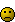12/1/2020 08:46If a,b are roots of quadratic equation Ax^2+Bx+C+D=0
find a^4+b^4 if B=C+D.

B^4-4B^3+2B^212/1/2020 14:25If a,b are roots of quadratic equation Ax^2+Bx+C+D=0find a^4+b^4 if B=C+D.B^4-4B^3+2B^2得B無A既我既問題係點解A會=1? 12/1/2020 14:44If a,b are roots of quadratic equation Ax^2+Bx+C+D=0find a^4+b^4 if B=C+D.My instinct is to find an expression for a^4+b^4 in terms of ab and a+ba^4+b^4=(a+b)^4 - (4a^3)(b) - (6a^2)(b^2) - (4a)(b^3)=(a+b)^4 - 6(ab)^2 - 4(ab)(a^2+b^2)=(a+b)^4 - 6(ab)^2 - 4(ab)((a+b)^2-2ab))and substitute the corresponding coefficients Although there could be a quicker way.a+b同ab係咩先OK. I will play along.a+b = -B/A, ab = B/ASubstituting into the obtained result, yields,a^4+b^4 = (B/A)^4 - 4(B/A)^3 + 2(B/A)^2 12/1/2020 14:55If a,b are roots of quadratic equation Ax^2+Bx+C+D=0find a^4+b^4 if B=C+D.B^4-4B^3+2B^2得B無A既我既問題係點解A會=1?我諗過了x^2+(-a-b)x+ab=0---(1)Ax^2+Bx+C+D=0---(2)From (2): x^2+(B/A)x+(B/A)=0---(3)Compare (1) and (3)B/A=-a-b=ab結論a+b,ab inversely proportional to A。 12/1/2020 14:56

快速回覆 - 輸入以下項目